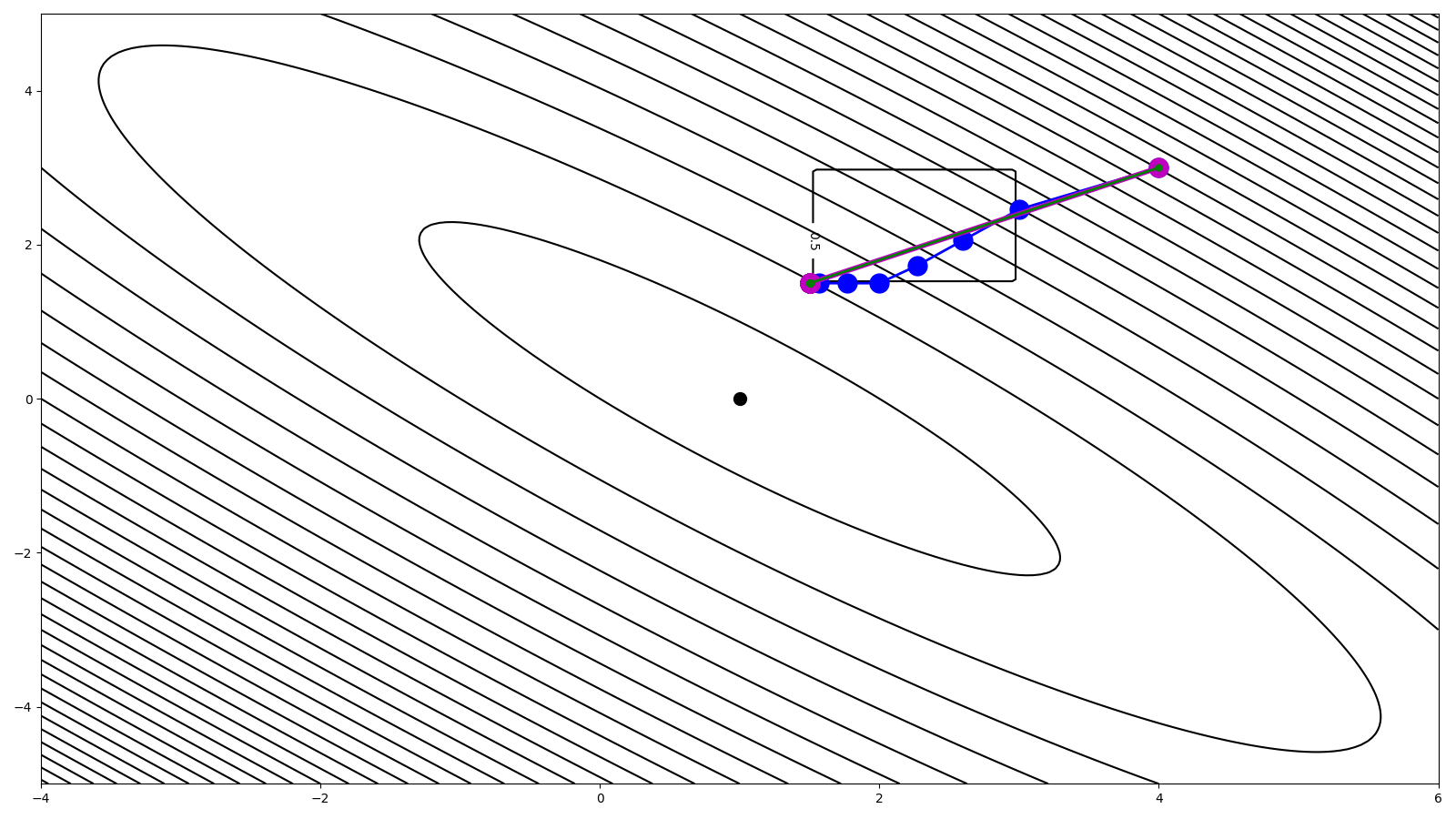# Quadratic program with box constraints#

This tutorial shows how we can use some of PyProximal solvers to solve a quadratic function with a box constraint:

$\mathbf{x} = \argmin_\mathbf{x} \frac{1}{2} \mathbf{x}^T \mathbf{A} \mathbf{x} + \mathbf{b}^T \mathbf{x} \quad s.t. \quad \mathbf{x} \in \mathcal{I}_{\operatorname{Box}}$

More specifically we will consider both the pyproximal.optimization.primal.ProximalGradient algorithm with and without back-tracking.

In the literature you may find that problem of this kind can be solved by the so-called Projected Gradient Descent (PGD) algorithm: this is a edge case of a Proximal gradient solver when used with a constraint that admits a proximal (instead of a soft regularizer).

import numpy as np
import matplotlib.pyplot as plt
import pylops

import pyproximal

plt.close('all')


Let’s start defining the terms of the quadratic functional

m = np.array([1, 0])
G = np.array([[10., 9.],
[9., 10.]])
d = np.dot(G, m)


We can now compute the functional within a grid, which we will show together with the evolution of the solution from the proximal gradient algorithm cost function grid

nm1, nm2 = 201, 201
m_min, m_max = (m - 5, m - 5), (m + 5, m + 5)
m1, m2 = np.linspace(m_min, m_max, nm1), \
np.linspace(m_min, m_max, nm2)
m1, m2 = np.meshgrid(m1, m2, indexing='ij')
mgrid = np.vstack((m1.ravel(), m2.ravel()))
J = 0.5 * np.sum(mgrid * np.dot(G, mgrid), axis=0) - np.dot(d, mgrid)
J = J.reshape(nm1, nm2)


We can now define the upper and lower bounds of the box and again we create a grid to display alongside the solution

lower = 1.5
upper = 3
indic = (mgrid > lower) & (mgrid < upper)
indic = indic.reshape(nm1, nm2) & indic.reshape(nm1, nm2)


We can now define both the quadratic functional and the box

l2 = pyproximal.L2(Op=pylops.MatrixMult(G), b=d, niter=2)
ind = pyproximal.Box(lower, upper)


We are now ready to solve our problem. All we need to do is to choose an initial guess for the proximal gradient algorithm

def callback(x):
mhist.append(x)

m0 = np.array([4, 3])

mhist = [m0,]
tau=0.0005,
x0=m0, epsg=1.,
niter=10,
callback=callback)
mhist_slow = np.array(mhist)


Provided we can estimate the spectral radius (i.e., max eigenvalue) of our operator G, we can choose an optimal step and improve our convergence speed.

L = np.max(np.linalg.eig(G)) # max eigenvalue of G
tau_opt = 1. / L
mhist = [m0,]
tau=tau_opt, x0=m0,
epsg=1., niter=10,
callback=callback)
mhist_opt = np.array(mhist)


Alternatively we can use back-tracking to adaptively find the best step at each iteration

mhist = [m0,]
x0=m0, epsg=1.,
niter=10,
niterback=4,
callback=callback)
mhist_back = np.array(mhist)


Finally let’s visualize the different trajectories and final solutions

fig, ax = plt.subplots(1, 1, figsize=(16, 9))
cs = ax.contour(m1, m2, J, levels=40, colors='k')
cs = ax.contour(m1, m2, indic, colors='k')
ax.clabel(cs, inline=1, fontsize=10)
ax.plot(m, m, '.k', ms=20)
ax.plot(m0, m0, '.r', ms=20)
ax.plot(mhist_slow[:, 0], mhist_slow[:, 1], '.-b', ms=30, lw=2)
ax.plot(mhist_opt[:, 0], mhist_opt[:, 1], '.-m', ms=30, lw=4)
ax.plot(mhist_back[:, 0], mhist_back[:, 1], '.-g', ms=10, lw=2)
plt.tight_layout()Total running time of the script: (0 minutes 0.360 seconds)

Gallery generated by Sphinx-Gallery